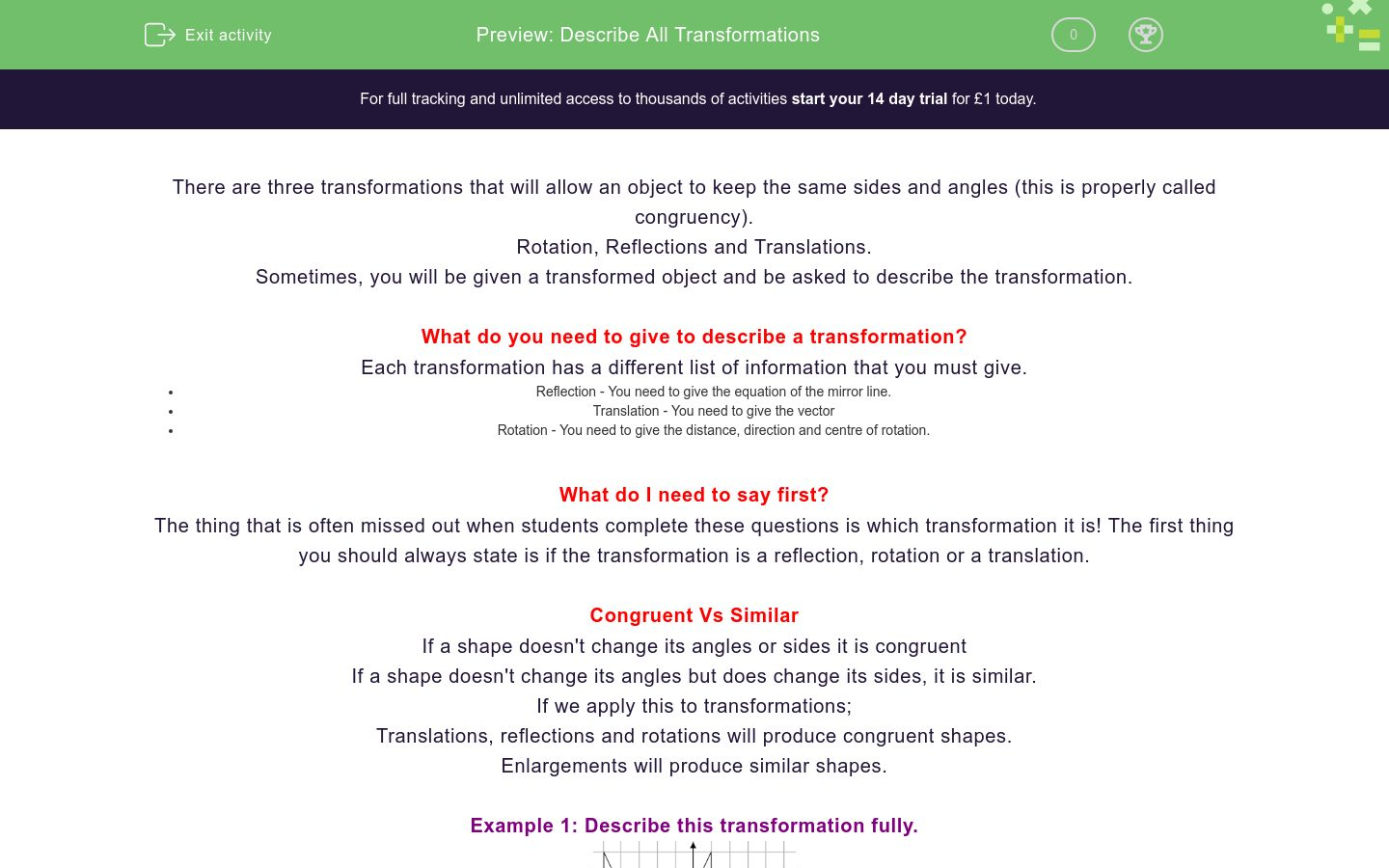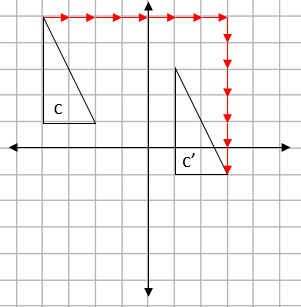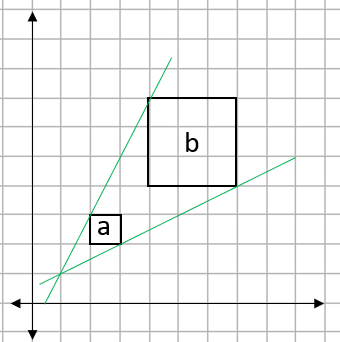# Describe All Transformations

In this worksheet, students practise describing all transformations.Key stage:  KS 4

GCSE Subjects:   Maths

GCSE Boards:   AQA, Eduqas, Pearson Edexcel, OCR

Curriculum topic:   Geometry and Measures, Congruence and Similarity

Curriculum subtopic:   Properties and Constructions, Plane Isometric Transformations

Difficulty level:### QUESTION 1 of 10

There are three transformations that will allow an object to keep the same sides and angles (this is properly called congruency).

Rotation, Reflections and Translations.

Sometimes, you will be given a transformed object and be asked to describe the transformation.

What do you need to give to describe a transformation?

Each transformation has a different list of information that you must give.

• Reflection - You need to give the equation of the mirror line.
• Translation - You need to give the vector
• Rotation - You need to give the distance, direction and centre of rotation.

What do I need to say first?

The thing that is often missed out when students complete these questions is which transformation it is! The first thing you should always state is if the transformation is a reflection, rotation or a translation.

Congruent Vs Similar

If a shape doesn't change its angles or sides it is congruent
If a shape doesn't change its angles but does change its sides, it is similar.

If we apply this to transformations;

Translations, reflections and rotations will produce congruent shapes.

Enlargements will produce similar shapes.

Example 1: Describe this transformation fully.Step 1: Decide which transformation this is.

a to a' is flipped. this means that the transformation is a reflection.

Step 2: Give the information we need.

For a reflection, we need a mirror line. This will always be exactly half way between the two shapes.This mirror line goes through the x axis at -2. This means the mirror line is x = -2

Example 2: Describe this transformation fully.Firstly, we can see that this is a rotation.The easiest way to find the centre of rotation is to trace the original shape and just try some points, when you find the one that works, that is the centre.

This transformation is described by...

Distance: 90°

Direction: Clockwise

Centre: (0,0)

Example 3: Describe this transformation fully.We can see that this is a translation.To describe a translation. We have to give a vector.

We can see from the arrows that are drawn that this is a movement of 7 to the right and 6 down.

This gives the vector...

 7 -6

Example 4: Shape A is enlarged to shape B. Find the scale factor and centre of enlargement.Finding the Scale Factor.

All we have to do here is to look at one of the pairs of sides. If we use the top of each shape, shape A is 1cm long and shape B is 3 cm long.

This gives a scale factor of 3.

Finding the centre of enlargement.

To find this, we join up two pairs of matching corners with striaght lines and see where they join up.We can see here that the two lines join up at the point (1,1). This is the centre of enlargement.

Match the transformation with the word that describes it best.

## Column B

Rotation
Slide/Move
Reflect
Turn
Translate
Flip
Enlargement
Change size

What type of transformation is shown here? Select all that applyReflection

Rotation

Translation

What is the equation of the mirror line for this reflection?What type of transformation is shown here? Select all that applyReflection

Rotation

Translation

Describe this rotation fully.Reflection

Rotation

Translation

What type of transformation is shown here? Select all that applyReflection

Rotation

Translation

Give the vector for this translation.a b

 Value a b

Shape a is enlarged around the point (0,0). What is the SF of the enlargement?Shape a is enlarged to shape a'. Find the SF and centre of the enlargement.Complete the following sentances...

• Question 1

Match the transformation with the word that describes it best.

## Column B

Rotation
Turn
Reflect
Flip
Translate
Slide/Move
Enlargement
Change size
EDDIE SAYS
These words are really useful command words. For example: You can easily spot if a shape has been turned, this means it has been rotated.
• Question 2

What type of transformation is shown here? Select all that applyReflection
Translation
EDDIE SAYS
This could either be a reflection as you could say the shape has flipped over, but you could also say that it has been slid downwards. It could also be a translation.
• Question 3

What is the equation of the mirror line for this reflection?y=2
EDDIE SAYS
The mirror line is always slap bang in the middle of the two shapes. For this question, it is a straight horizontal line that goes through the y - axis at 2
• Question 4

What type of transformation is shown here? Select all that applyRotation
EDDIE SAYS
This shape has been turned, this means it has to be a...
• Question 5

Describe this rotation fully.EDDIE SAYS
Remember that there are two different rotations we could state. It's either 90 CW or 270 ACW
• Question 6

What type of transformation is shown here? Select all that applyTranslation
EDDIE SAYS
This shape has been slid across and up, this means it is a...
• Question 7

Give the vector for this translation.a b

 Value a b
EDDIE SAYS
This shape has been moved 5 to the right and 3 up, this gives the vector...
• Question 8

Shape a is enlarged around the point (0,0). What is the SF of the enlargement?2
EDDIE SAYS
If we count the length of the sides a and a', we see that a' is twice the length of a.
• Question 9

Shape a is enlarged to shape a'. Find the SF and centre of the enlargement.EDDIE SAYS
Comparing the sides shows that a' is three times longer than a Drawing lines back through the corners will give the centre of enlargement
• Question 10

Complete the following sentances...

EDDIE SAYS
These are command words that you really must know. If a shape doesn't change its angles or sides it is congruent If a shape doesn't change its angles but does change its sides, it is similar.
---- OR ----